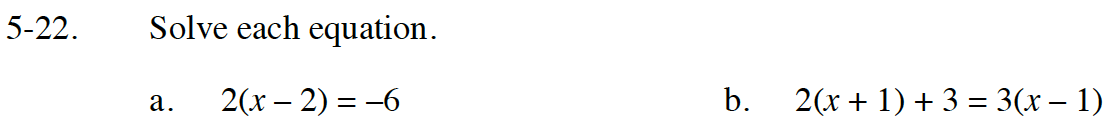Home > INT1 > Chapter Ch5 > Lesson 5.1.2 > Problem5-22

5-22.
1. Solve each equation. Homework Help ✎

1. 2(x – 2) = –6

2. 2(x + 1) + 3 = 3(x – 1)Option 1: Distribute the 2 into the (x - 2) term.
Option 2: Divide both sides of the equation by 2.

x = 8